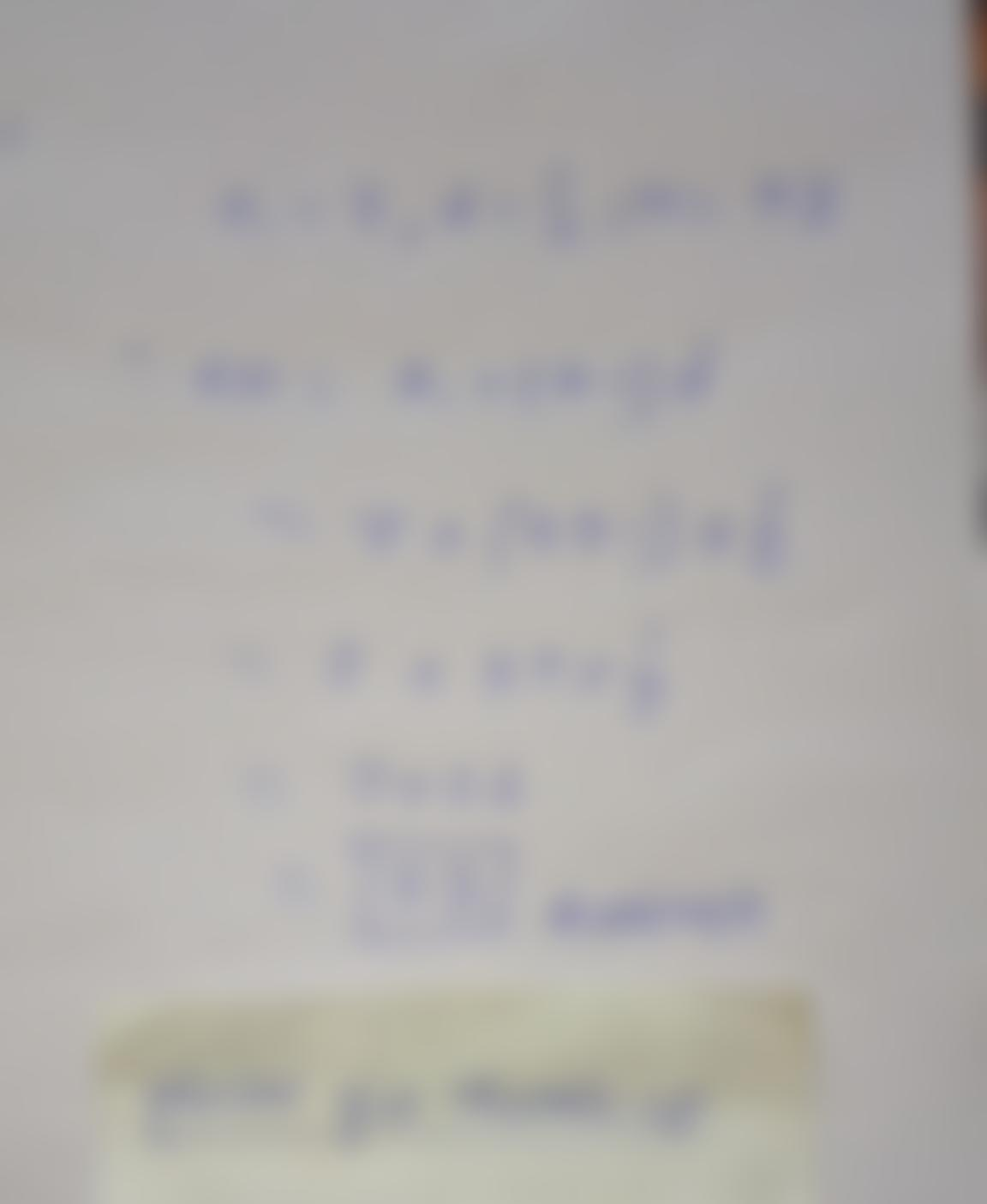Question:

Student - nocccd.edu X MyLab and Mastering X Course Home X P Do Homework - Section 9.2 X k > G mathxl.com/Student/PlayerHomeworkStudent - nocccd.edu X MyLab and Mastering X Course Home X P Do Homework - Section 9.2 X k > G mathxl.com/Student/PlayerHomework.aspx?homeworkld=615176564&questionld=2&flushed=false&cld=6644854&centerwin= Fall 2021 Math 143 College Algebra = Homework: Section 9.2 Question 7, 9.2.21 Part 1 of 2 HW Score: 4. O Points: 0 Find the nth term of the arithmetic sequence {an} whose initial term a and common difference d are given below. What is the fifty-third term? Next question 1 2, = 7; d = 2 What is the nth term of the arithmetic sequence {a,}? an (Simplify your answer. Use integers or fractions for any numbers in the expression.)